# Getting to the Point

## OBJECTIVE: To learn how to convert fractions to decimals

In previous lessons, you learned how to work with fractions. Wearing Uniforms: https://www.geogebra.org/m/ceyu3wpa Mixing 'Em Up: https://www.geogebra.org/m/hzx95nwa Reducing, Reusing, Recycling: https://www.geogebra.org/m/gcxtdncg Doing the Flip: https://www.geogebra.org/m/pqym69rc In this lesson, you're going to learn how to convert FRACTIONS to DECIMALS and subsequently perform operations on decimals. Fractions and decimals are simply two ways of expressing the relationship of a part to the whole. The applet below will show you graphically how fractions and decimals are related using a bar model. Interact with the applet for a few minutes. Move the sliders to generate fractions and observe how they get converted to decimals. The arithmetic process will be discussed later.

## HOW TO CONVERT FRACTIONS TO DECIMALS

A fraction is simply an implied division with the FRACTION BAR (also called DIVISION BAR) functioning as the DIVISION BOX in long division. So to convert a fraction into a decimal, just do the division and place the DECIMAL POINT in its proper place. Examples: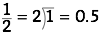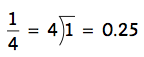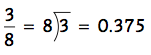One convenient method to change fractions to decimals without doing long division is to come up with an EQUIVALENT FRACTION whose denominator is a power of 10 and then move the decimal point of the numerator to the left the same number of times as there are zeros in the denominator. The denominator is then removed. Of course, this method will only work for fractions whose denominator is a factor of powers of 10. Examples: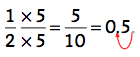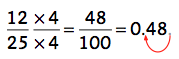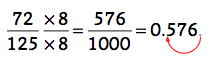One more thing, dividing the numerator by the denominator doesn't always end up as a TERMINATING DECIMAL (one that ends). Sometimes, you'll get a REPEATING DECIMAL (one that repeats a certain number of digits). In the latter case, a horizontal bar is placed over the top of the digits that repeat. Examples: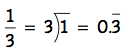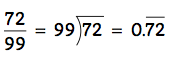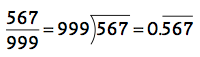## Use the applet below for practice.

Below is a set of problems that require you to convert fractions to decimals.

## In this lesson, you learned how to convert fractions to decimals.

In future lessons, you'll do more work on fractions and decimals. Hope you had FUN today.